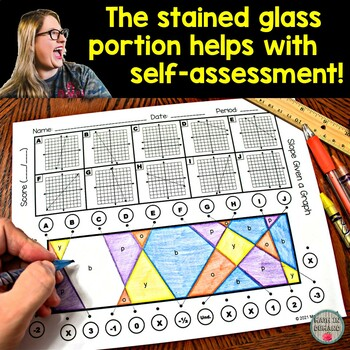# Slope Stained Glass Activity 4 Worksheets Graph, Table, 2 Points, Standard Form

Rated 4 out of 5, based on 1 reviews
1 Rating;
8th - 9th
Subjects
Resource Type
Formats Included
• PDF
Pages
Report this resource to TPT
##### Also included in
1. This is a bundle for all of my 8th Grade Math Stained Glass Activities. This bundle includes the following math concepts:1.) Solving Two-Step Equations2.) Solving Two-Step Equations with Fractions3.) Solving Two-Step Equations with Parentheses4.) Solving Equations with Variables on Both Sides5.) Slo
Price \$22.50Original Price \$25.00Save \$2.50

### Description

There are 4 worksheets on slope given a graph, table, two points, and standard form. Each worksheet contains 10 problems. The 4 worksheets are: (1) Slope Given a Graph, (2) Slope Given a Table, (3) Slope Given 2 Points, and (4) Slope Given Standard Form.

These worksheets help with self-assessment due to creating a stained glass look. Students will use a ruler or straight edge to connect the letter of the question to its answer. After connecting 10 lines, students should see a letter in each section. Students will color each section according to the color code.

Total Pages